# Introduction

This document provides examples for using the wildlifeDI package for investigating dynamic interaction patterns in wildlife telemetry data. Dynamic interaction can be defined as the inter-dependency in the movements of two individuals. Traditional methods for measuring dynamic interaction treat telemetry data as a spatial point-pattern, and examine interactions based on distances between paired points (i.e., those simultaneous in time) vs. expectations based on the distribution of distances between all points. Newer methods attempt to measure dynamic interaction as the cohesiveness (or similarity) in corresponding movement segments. Several measures of dynamic interaction are included in this suite of tools. In the following sections I will outline the functionality of each method, along with some guidelines and tips for where and when to use each method, and how to interpret results. These tools assume one has a working knowledge of the adehabitat package and classes (i.e., ltraj objects) used for working with movement data in R (Calenge 2006).

## Some Terminology

Before we go any further it is imperative that we clarify some terminology that will be used in the following explanations (Table 1).

Table 1: Terminology used in describing dynamic interaction methods.

Symbol Explanation
$$\alpha$$ or $$\beta$$ Individuals (telemetry data)
fix A telemetry record (spatial location and time stamp)
segment The vector connecting two consecutive fixes
T$$_{\alpha\beta}$$ Temporally simultaneous fixes, based on a time threshold $$t_c$$
S$$_{\alpha\beta}$$ Spatially proximal fixes, based on a distance threshold $$d_c$$
ST$$_{\alpha\beta}$$ Spatially proximal and temporally simultaneous fixes, based on $$d_c$$ and $$t_c$$

## Data

We examine a GPS telemetry dataset representing the movement of two deer over a one week interval. These data are provided as part of the wildlifeDI package, and are a subset of the data set explored in the case study in Long et al. (2014). For more information on how the deer data was collected or for citation please see the papers by Webb et al. (2009, 2010).

library(wildlifeDI)
data(deer)
deer
##
## *********** List of class ltraj ***********
##
## Type of the traject: Type II (time recorded)
## * Time zone unspecified: dates printed in user time zone *
## Irregular traject. Variable time lag between two locs
##
## Characteristics of the bursts:
##   id burst nb.reloc NAs          date.begin            date.end
## 1 37    37      551   0 2005-03-07 19:03:00 2005-03-13 18:47:00
## 2 38    38      567   0 2005-03-07 19:02:00 2005-03-13 18:47:00
##
##
##  infolocs provided. The following variables are available:
##  "pkey"

As you can see, there are two individuals contained in this dataset, which are named based on their ids: id = 37 and id = 38. The deer data represent the movement of these two individual deer over a one week period, with GPS fixes attempted at a 15 minute interval.

In order to utilize the tools, each individuals movement trajectory needs to be stored as a separate ltraj object, which is simple to do. Here we simply extract the 1st and 2nd bursts in order to extract separate ltraj objects for each individual.

deer37 <- deer
deer37
##
## *********** List of class ltraj ***********
##
## Type of the traject: Type II (time recorded)
## * Time zone unspecified: dates printed in user time zone *
## Irregular traject. Variable time lag between two locs
##
## Characteristics of the bursts:
##   id burst nb.reloc NAs          date.begin            date.end
## 1 37    37      551   0 2005-03-07 19:03:00 2005-03-13 18:47:00
##
##
##  infolocs provided. The following variables are available:
##  "pkey"
deer38 <- deer
deer38
##
## *********** List of class ltraj ***********
##
## Type of the traject: Type II (time recorded)
## * Time zone unspecified: dates printed in user time zone *
## Irregular traject. Variable time lag between two locs
##
## Characteristics of the bursts:
##   id burst nb.reloc NAs          date.begin            date.end
## 1 38    38      567   0 2005-03-07 19:02:00 2005-03-13 18:47:00
##
##
##  infolocs provided. The following variables are available:
##  "pkey"

## Checking for temporal overlap

Before embarking on analysis of dynamic interaction, it is worthwhile to check whether two telemetry datasets overlap temporally. This can be easily done using the checkTO function. The function tells us first if the two datasets overlap temporally (a necessary condition for spatial-temporal interaction), then it gives us the timings of the start and end of the overlap period.

checkTO(deer37,deer38)
## $TO ##  TRUE ## ##$TOstart
##  "2005-03-07 19:03:00 EST"
##
## $TOend ##  "2005-03-13 18:47:00 EST" Here we can clearly see that the two deer overlap for essentially the whole period, as these data were hand picked for this purpose. However, this will not always be the case, and thus checkTO can be a useful function for identifying if, and when, two telemetry datasets overlap temporally. ## Static interaction analysis Static interaction can be defined broadly as the spatial overlap of two individual home ranges, or more recently, as the volume of intersection between two individual utilization distributions (Macdonald et al. 1980, Millspaugh et al. 2004). It is often useful to examine static interaction to investigate the potential for dynamic interactions to exist. Here we investigate the simpler case of proportion of home range overlap, to test for the potential for dynamic interaction between deer37 and deer38. The proportion of overlap is calculated simply as: $\text{SI} = \frac{HR_\alpha \cap HR_\beta}{HR_\alpha \cup HR_\beta}$ where HR refers to the corresponding home range area. To compute individual home ranges we will simply use the minimum convex polygon. library(sf) #convert ltraj to sf points to compute mcp polygon pts37 <- ltraj2sf(deer37) pts38 <- ltraj2sf(deer38) #compute mcp polygons hr37 <- st_convex_hull(st_union(pts37)) hr38 <- st_convex_hull(st_union(pts38)) #plot plot(hr38) plot(hr37,border="red",add=T)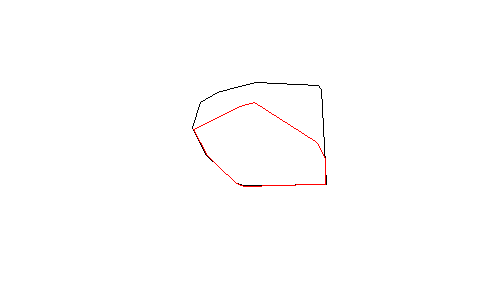#Compute SI index st_area(st_intersection(hr37,hr38))/st_area(st_union(hr37,hr38)) ##  0.6435784 Here we can see there is substantial overlap in home ranges between these two individuals, and thus some would suggest that this may be evidence of likely dynamic interaction, which is what we will explore further. NOTE: The MCP home ranges we have computed here (saved as the objects hr37 and hr38) will be used in later analysis. ## Obtaining Simultaneous Fixes - T$$_{\alpha\beta}$$ Measurement of dynamic interaction often requires the identification of those fixes that are deemed to be simultaneous in time (T$$_{\alpha\beta}$$) based on some time tolerance threshold - $$t_c$$. As this is rarely the case in real datasets, the function GetSimultaneous was developed to extract simultaneous fixes from two movement datasets. The tolerance parameter ($$t_c$$) can be used to allow the times to deviate slightly and still be considered simultaneous. Note that that ltraj objects, despite displaying date-time formats, measure times in seconds and thus the $$t_c$$ argument is given in seconds. The documentation for the GetSimultaneous function tells us to pass in two trajectories, and a tc argument. In this example we will use 7.5 minutes as $$t_c$$, which is 1/2 the sampling interval (which is generally a good starting point) of 15 minutes. This means that any two fixes that are within 7.5 minutes of each-other are deemed simultaneous. The result of the GetSimultaneous function is a list with two ltraj objects which can be extracted. deers <- GetSimultaneous(deer37,deer38,tc=7.5*60) deer37.sim <- deers deer38.sim <- deers deer37.sim ## ## *********** List of class ltraj *********** ## ## Type of the traject: Type II (time recorded) ## * Time zone unspecified: dates printed in user time zone * ## Irregular traject. Variable time lag between two locs ## ## Characteristics of the bursts: ## id burst nb.reloc NAs date.begin date.end ## 1 37 37 546 0 2005-03-07 19:03:00 2005-03-13 18:47:00 ## ## ## infolocs provided. The following variables are available: ##  "pkey" deer38.sim ## ## *********** List of class ltraj *********** ## ## Type of the traject: Type II (time recorded) ## * Time zone unspecified: dates printed in user time zone * ## Irregular traject. Variable time lag between two locs ## ## Characteristics of the bursts: ## id burst nb.reloc NAs date.begin date.end ## 1 38 38 546 0 2005-03-07 19:02:00 2005-03-13 18:47:00 ## ## ## infolocs provided. The following variables are available: ##  "pkey" As you can now see, these trajectories have an equal number (nb.reloc = 546) of simultaneous fixes based on the supplied $$t_c$$ value of 7.5 minutes. Also, recall that in the original data deer37 and deer38 contained 551 and 567 fixes, respectively. However, as we just demonstrated only 546 of these fixes were deemed to be simultaneous. The Function GetSimultaneous is used internally with most methods, so it is generally not used on its own, however it can be useful to obtain simultaneous fixes for other analyses, which is why it is included here. # Measuring Dynamic Interaction ## Global Analysis Here I have defined dynamic interaction analysis as being one of either global or local. Some global statistics have a local alternative so will be discussed in both sections. Global analysis generates a single output statistic for the entire dataset (i.e., pair of individuals), while local analysis generates an output statistic at every single point in the dataset, defined here as simultaneous fixes. ### Prox - Proximity analysis Proximity analysis (see Bertrand et al. 1996) can be a useful, simple way to examine attraction in wildlife telemetry studies. Of interest is determining the proportion of the T$$_{\alpha\beta}$$ (simultaneous fixes) that are ST$$_{\alpha\beta}$$ (simultaneous and proximal fixes) based on the given distance threshold $$d_c$$. It is simply calculated as: $\text{Prox} = \frac{T_{\alpha\beta}}{ST_{\alpha\beta}}$ Further, it can be useful to measure the variability in proximity through time. Thus, simply creating a time-series graphic of $$d_{\alpha\beta}$$ can be of interest. The function Prox can be used to implement proximity analysis in R. It requires that the user define $$t_c$$ to be passed to the function GetSimultaneous. The Prox function also requires the user to pass in an appropriate $$d_c$$ value for determining the spatial threshold at which fixes are proximal. Throughout this analysis we use a $$t_c$$ of 7.5 minutes and $$d_c$$ of 50 meters. Note: the spatial coordinates of the deer data are stored in UTM format making meters the appropriate spatial unit. Prox(deer37, deer38, tc=7.5*60, dc=50) ##  0.4139194 Interpretation: Here the Prox statistic is 0.4139, an indication that there is definitely attraction by this pair. A Prox value of 0.4139 means that $$41.39\%$$ of the simultaneous fixes were within the defined distance threshold $$d_c$$ (50 m) of each other. ### Ca - Coefficient of association The coefficient of association (Ca; Cole 1949, Bauman 1998) statistic measures the proportion of fixes that are ST$$_{\alpha\beta}$$ based on the given distance threshold $$d_c$$. It is simply calculated as: $\text{Ca} = \frac{2AB}{A+B}$ where AB is the number of ST$$_{\alpha\beta}$$ fixes, and A and B are the number of fixes in $$\alpha$$ and $$\beta$$ respectively. It has been suggested in the literature that a cut-off of 0.5 can be used to identify attraction (Ca $$> 0.5$$) and avoidance (Ca $$< 0.5$$). The function Ca can be used to implement the Ca statistic in R. Again, we use threshold values of $$t_c$$ = 7.5 minutes and $$d_c$$ = 50 meters. Ca(deer37, deer38, tc=7.5*60, dc=50) ##  0.4042934 Interpretation: Here the Ca statistic is 0.4043, an indication that there is moderate attraction by this pair. However, the Ca value is not $$> 0.5$$ and we would not expect attraction based on the literature which suggests only Ca $$> 0.5$$ as attraction. However, based on the Prox index, we know that some attraction behaviour occurs, and Ca corroborates this evidence with a Ca = 0.4043 which is near 0.5. ### Don - Doncaster’s non-parametric test of interaction Doncaster’s (1990) non-parametric test for interaction follows from Knox’s (1964) test for space-time clustering. Essentially, Don is used to examine differences in the the distribution of distances between T$$_{\alpha\beta}$$ fixes, and the set of $$n^2 - n$$ permutations of non-T$$_{\alpha\beta}$$ fixes. The cumulative distribution of the T$$_{\alpha\beta}$$ fix distances can be compared graphically with the cumulative distribution of the $$n^2 - n$$ permuted distances. This can be useful, for example, to determine a suitable distance threshold - $$d_c$$ by identifying where the T$$_{\alpha\beta}$$ plot is below the expected line based on the $$n^2 - n$$ permutations. Upon selecting a suitable $$d_c$$ value, a contingency table can be constructed, identifying the number of T$$_{\alpha\beta}$$ and non-T$$_{\alpha\beta}$$ (termed unpaired) fix distances that are above and below the threshold $$d_c$$. A $$\chi^2$$ test with 1 d.f. can be used to examine statistically the difference in T$$_{\alpha\beta}$$ and non-T$$_{\alpha\beta}$$ distances above and below $$d_c$$. The function Don computes Doncaster’s non-parametric test. It requires a time threshold for simultaneous fixes ($$t_c$$), along with a value for $$d_c$$ in appropriate units. The output presents the cumulative distribution plot, the contingency table of distances, and the $$\chi^2$$ test result. Significant $$\chi^2$$ values are indicative of attraction, while non-significant results suggest indifference. Don(deer37,deer38, tc=7.5*60, dc=50)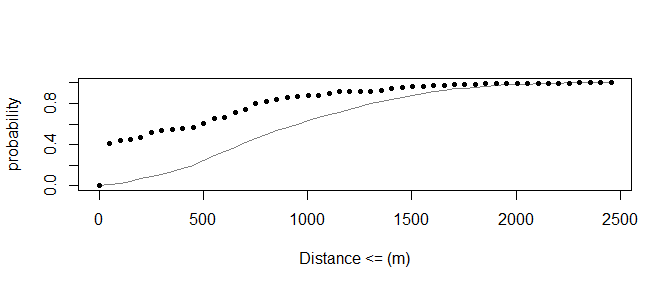##$conTable
##            below.crit above.crit Totals
## Paired            226        320    546
## Non-Paired       3925     293637 297570
## Totals           4159     293957 298116
##
## $p.value ##  0 Interpretation: The graph of the count of observed (black dots) vs. expected (grey line) fix distances, for a range of distance intervals, suggests that there may be some attraction at lower distance intervals, due to the observed values being to the left of the expected line. The Don plot can often be used to examine differences in the effect of the $$d_c$$ parameter and the range at which attraction behaviour may occur. The significant p-value of 0 suggests significant attraction occurs, an expected result given the Prox and Ca statistics. Also, we can reaffirm, using the contingency table, that 226 paired (simultaneous) fixes are within the defined distance threshold ($$d_c$$ = 50 m), and 320 paired (simultaneous) fixes are not within the defined distance threshold. ### Lixn - Test for spatial and temporal interaction Minta (1992) introduced three statistics (L$$_{AA}$$, L$$_{BB}$$, and L$$_{ixn}$$) for examining spatial and temporal interactions between animals. All three of the statistics require the delineation of a ‘shared-area’ between the two animals. If home ranges can be estimated, the shared-area can be defined as the spatial intersection between the individual home ranges, defined a priori. In this case, the L$$_{ixn}$$ statistic is computed using the "spatial" method. With the "spatial" method home ranges are divided (through a spatial intersection) into three areas: belonging to $$\alpha$$ only, belonging to $$\beta$$ only, and shared by $$\alpha$$ and $$\beta$$ (also termed the overlap zone). If home ranges cannot be estimated, but some overlap zone is known, L$$_{ixn}$$ can still be computed. In this case, one should use the "frequency" method. The known overlap zone may be some area known to be associated with both individuals (e.g., a natural reserve site, or an important feeding ground). Note: with modern telemetry datasets, home ranges are easily estimated using one of a host of methods, and thus the "spatial" method is usually the appropriate choice with Lixn. The first two statistics computed (L$$_{AA}$$ and L$$_{BB}$$), represent spatial interaction statistics. They examine how each individual utilizes the shared area. The number of fixes contained in each area (i.e., $$\alpha$$’s area, $$\beta$$’s area and the shared area), are tested against expectations representing the probability of finding the animal in a given area derived from either the overlap areal percentages (method = "spatial") or based on the proportions of all fixes contained in each area (method = "frequency"). For more information on the formulation of each calculation see Minta (1992). Essentially, L$$_{AA}$$ (respectively L$$_{BB}$$) tests how each individual uses their independent and shared home range areas. When L$$_{AA}$$ $$\simeq 0$$, $$\alpha$$ uses the shared area randomly, while L$$_{AA} > 0$$ indicates spatial attraction to the shared area, and L$$_{AA} < 0$$ indicates spatial avoidance of the shared area. L$$_{BB}$$ is interpreted identically with respect to $$\beta$$. Using the same expectation probabilities derived for use with L$$_{AA}$$ and L$$_{BB}$$, the L$$_{ixn}$$ statistic is a function of the ratio of simultaneous use (and avoidance) of the shared area and solitary use (and avoidance) of the shared area. Thus, the L$$_{ixn}$$ statistic is a measure of the simultaneity of use of the shared area. Note that this does not directly account for the actual distance between the two individuals, so when the shared area is large, the interpretation of interaction may be different from when the shared area is small. When L$$_{ixn}$$ $$\simeq 0$$ it suggests both individuals use the shared area randomly. L$$_{ixn} > 0$$ indicates use of the shared area that is simultaneous (i.e., attraction), while L$$_{ixn} < 0$$ indicates use of the shared area that is solitary (i.e., avoidance). The Minta (1992) statistics (L$$_{AA}$$, L$$_{BB}$$, and L$$_{ixn}$$) are all drawn from observed and expected values taken from a 2x2 contingency table. Thus, a $$\chi^2$$ test with 1 d.f. can be used to make statistical inferences on the (L$$_{AA}$$, L$$_{BB}$$, and L$$_{ixn}$$) values. As with previous methods, the user is required to submit a value for $$t_c$$ to be passed to the function GetSimultaneous internally. If method="spatial" the user is required to input the home-ranges, stored as a SpatialPolygons* object for each individual. If method="frequency" the user is required to pass in the overlap zone (OZ), stored as a SpatialPolygons* object. Here we use the home ranges, previously calculated in section 2.1 (i.e., the objects hr37 and hr38), which will be passed into the Lixn function. Along with the L$$_{AA}$$, L$$_{BB}$$, and L$$_{ixn}$$ statistics and their associated p-values, the function returns contingency tables for the expected probabilities, observed values, and odds depicting the simultaneous and solitary use of the shared area by each individual. Lixn(deer37, deer38, method='spatial', tc=7.5*60, hr1=hr37, hr2=hr38) ##$pTable
##           A           b
## B 0.6423984 0.003342149
## b 0.3524259 0.001833535
##
## $nTable ## A b ## B 481 10 ## b 55 0 ## ##$oTable
##           A        b
## B 1.3713489 5.480012
## b 0.2858263 0.000000
##
## $Laa ##  -1.277045 ## ##$p.AA
##  1.879819e-05
##
## $Lbb ##  1.588743 ## ##$p.BB
##  0
##
## $Lixn ##  -1.436156 ## ##$p.IXN
##  0.0004248804

interpretation: Interpretation of the results is dependent on the derivation of home ranges (here MCP). L$$_{AA}$$ is negative adn significant, whereas L$$_{BB}$$ is $$> 0$$ and significant (p-values $$< 0.05$$). The positive and significant values suggest that deer38 is attracted to the shared-area (the overlap area of the home ranges). The value for L$$_{ixn}$$ is negative, suggesting no evidence of simultaneous use of the shared area, and this value is significant. The negative L$$_{ixn}$$ result here is a funciton of the MCP home ranges and the fact that using this home range method, deer37s home range is nearly encompasssed by deer 38s. Making this particular example difficult to interpret.

### Cs - Coefficient of sociality

The coefficient of sociality (Cs; Kenward et al. 1993) incorporates the mean distances of T$$_{\alpha\beta}$$ fixes ($$D_O$$) and the mean distances of the $$n^2$$ permutations of all fixes ($$D_E$$) into a single statistic. $\text{Cs} = \frac{D_E - D_O}{D_E + D_O}$ Generally, the following interpretation of Cs has been suggested: Cs $$\simeq$$ 1 indicates attraction, while Cs $$\simeq$$ -1 indicates avoidance. However, because the observed values are paired, a Wilcox signed-rank test can be used to determine the significance of Cs, rather than relying on the more subjective interpretation.

The Cs statistic is calculated using the function Cs, which produces output giving the observed and expected fix distances, the Cs value, and one-sided p-values resulting from the Wilcox signed-rank test for significant attraction and avoidance.

Cs(deer37, deer38, tc=7.5*60)
## $Do ##  422.3163 ## ##$De
##  873.9819
##
## $Cs ##  0.3484272 ## ##$p.Attract
##  7.403068e-53
##
## $p.Avoid ##  1 interpretation: First, we see that the Cs statistic (Cs = 0.3484) is definitely above 0, although it is closer to 0 than 1. From here, we can use the significant test to aid our interpretation, we can see there is significant attraction (p.Attract < 0.05),but no evidence of significant avoidance (p.Avoid > 0.05). ### HAI - Half-weight association index The HAI (Atwood and Wells 2003) utilizes the shared area between the two individual home ranges (often termed the overlap zone). HAI is calculated in identical fashion to Ca, but HAI provides a more spatially localized approach, focusing only on the fixes within the shared area (overlap zone). The statistic takes the following form: $\text{HAI} = \frac{n}{n + \dfrac{a + b}{2}}$ where $$n$$ is the number of ST$$_{\alpha\beta}$$ fixes in the shared area based on user given thresholds for $$t_c$$ and $$d_c$$, and $$a$$ and $$b$$ are the number of solitary fixes, for $$\alpha$$ and $$\beta$$ respectively, in the shared area. Essentially, HAI tests ST$$_{\alpha\beta}$$ use of the shared area against solitary use of the shared area. This is useful, as interaction would not be expected outside of the shared area of the home ranges. When HAI $$\simeq 1$$ it is an indication of attraction, and when HAI $$\simeq 0$$ it is an indication of avoidance. The HAI statistic can be computed via the function HAI. Like Ca, HAI requires that the user input values for the thresholds $$t_c$$ and $$d_c$$, but also like Lixn that the user provide a polygon for defining the overlap zones. The overlap zone (OZ) must be a SpatialPolygons* object. The output is simply the value of the HAI statistic, which can be interpreted identically to Ca, that is a cut-off of 0.5 can be used to identify attraction (HAI $$> 0.5$$) and avoidance (HAI $$< 0.5$$). Below we use the package rgeos and the function gIntersection to compute the intersection between the two deer home ranges and delineate the overlap zone, stored as object oz. #compute overlap zone oz <- st_intersection(hr37, hr38) HAI(deer37, deer38, oz, tc=7.5*60, dc=50) ##  0.2619048 interpretation: Here we see that HAI is 0.2942, which suggests there is little evidence of attraction in the shared area of the home range (HAI $$< 0.5$$). In comparison with Ca = 0.4043, HAI is found to be lower here, which suggests there may have been a number of simultaneous fixes outside of the shared-area that were within the $$d_c$$ = 50m threshold. ### IAB - Interaction Statistic The IAB statistic (Benhamou et al. 2014) takes an alternative view on testing for dynamic interaction from telemetry data. It computes an index (IAB) analogous to the Bhattacharyya coefficient between the two animals. $\text{IAB}(t) = exp\left[ -0.5(D_{AB}(t)/\Delta)^2 \right]$ where $$D_{AB}$$ is the distance between two simultaneous ($$T_{\alpha\beta}$$) telemetry fixes. Instead of using a critical distance threshold, the IAB statistic uses a parameter ($$\Delta$$) that represents the point maximum slope of the distance effect function which measures the potential influence domain between the two animals. In the R function $$\Delta$$ is simply the dc parameter. Further, a novel simulation procedure is proposed for generating the expectation against which a statistical test is based. That is, a wrapped shifting method is used to maintain the serial correlation structure implicit to the movement data. At each shift, a sample statistic (termed MAB) is computed to generate the distribution of values for the test statistic. IAB(deer37, deer38, dc=50, tc=7.5*60) ##$IAB.obs
##  0.3986007
##
## $IAB.exp ##  0.01694352 ## ##$P.attract
##  0.001831502
##
## $P.avoid ##  1 Interpretation: Here we can see that the IAB test suggests significant attraction ($$p = 0.0018$$) and conversely no avoidance, which would be expected. The IAB statistic further corroborates evidence from the other indices that there is attraction between these two deer. ### Cr - Correlation coefficient The correlation coefficient (Cr) was proposed by Shirabe (2006) to measure the degree of correlation in movement data represented as a path as opposed to as points (that is, as n - 1 movement segments). The Cr statistic takes the form of a multivariate Pearson product-moment correlation coefficient (see Shirabe 2006 for more details on how Cr is computed). Essentially, Cr is based on computing differences in the simultaneous path segments between $$\alpha$$ and $$\beta$$. The differences are defined as deviations from the respective path mean vectors. Interpretation of the Cr statistic is similar to a typical correlation coefficient: Cr $$\simeq 1$$ indicates correlated movements, while Cr $$\simeq -1$$ indicates negatively correlated movements (e.g., repulsion), and Cr $$\simeq 0$$ indicates random movement, with respect to the other individual. It is important to note that Cr does not account for the distance between the two individuals at any point in its derivation, thus it is up to the analyst to infer whether the correlations measured are in fact meaningful. Cr(deer37, deer38, tc=7.5*60) ##  0.3706059 interpretation: A Cr value of 0.3706 indicates that there is some evidence for cohesive behaviour. Specifically, we interpret Cr like a correlation coefficient. It is difficult to know in this case if the slightly positive Cr value suggests that the two deer movements are correlated, but based on the result from Prox, we would expect this behaviour to occur from Thursday to Sunday. ### DI - Dynamic interaction index The global dynamic interaction index (DI) proposed by Long and Nelson (2013) is similar to the Cr statistic in that it uses path based analysis. The DI index attempts to measure cohesiveness in two independent components of movement: direction (often termed azimuth) and speed (generally measured using segment displacements). The DI index includes two main differences from the Cr statistic in its formulation; 1) DI does not depend on the respective path mean vectors, and 2) DI can disentangle the independent effects of correlation in direction and speed. $\text{di}_t = \left(1 - \left(\frac{\lvert d^\alpha_t - d^\beta_t \rvert}{d^\alpha_t + d^\beta_t}\right)^\delta\right) \times \cos\left(\theta^\alpha_t - \theta^\beta_t\right)$ $\text{DI} = \sum\limits^{n-1}_{t=1} \text{di}_t$ where $$d^\alpha_t$$ ($$\beta$$ respectively) are movement displacements for segment $$t$$, and $$\theta^\alpha_t$$ ($$\beta$$ respectively) are movement azimuths for segment $$t$$. The parameter $$\delta$$ is a scaling factor for the displacement component (denoted as $$\alpha$$ in Long and Nelson 2013). Calculation of DI is computed via the function DI. The DI function outputs the value of the DI (along with DI$$_{\theta}$$ and DI$$_d$$). DI(deer37, deer38, tc=7.5*60) ##$DI
##  0.1510688
##
## $DI.theta ##  0.1735282 ## ##$DI.d
##  0.5910381
##
## $P.positive ##  0.001834862 ## ##$P.negative
##  1

interpretation: Here we see the global DI value that is close to zero (DI=0.1511), suggesting there is little cohesion in the movements of the two deer. From the two other metrics, we can see that the cohesiveness in movement displacement (DI$$_d$$ = 0.591) is much higher than the cohesiveness in movement direction (DI$$_{\theta}$$ = 0.1735). The strong cohesiveness in movement displacement suggests that the two deer move at similar speeds at similar times, whether or not they are moving in the same direction (as evident by the low DI$$_{\theta}$$). The statistical test is taken from Benhamou et al. (2014) and the IAB index. Here we see that although DI is low, it is identified as significant based on the expectation from the permutations.

## Local Analysis

With local analysis, we wish to explore the spatial and temporal dynamics of dynamic interaction, that is to uncover temporal and spatial patterns in dynamic interaction not otherwise noticeable from global level analysis. Three of the above indices have a ‘local’ version that can be readily implemented through the wildlifeDI package. Local analysis should, in all cases, be considered as an exploratory analysis, and reported p-values and z-scores should be interpreted with this in mind.

### Proximity Analysis

Proximity analysis via the function Prox is easily extended to local analysis, in that we can compute the proximity (i.e., Euclidean distance) between each simultaneous fix. This is done in the function Prox by setting the option local = TRUE; the output is a dataframe for easy further manipulation.

prox.df <- Prox(deer37, deer38, tc=7.5*60, dc=50, local=TRUE)
plot(prox.df$date1,prox.df$prox,type="l")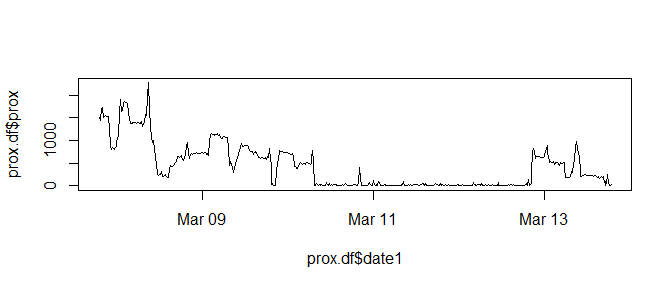Interpretation: We can use this time-series plot of proximity to examine the local-scale variation in proximity between the two deer. For instance, it appears the two deer remained close together from mid-day Thursday until around Sunday morning. Examining temporal variation in Prox can be useful for exploring temporal covariates associated with attraction behaviour.

### IAB index

Here I have also implemented a local version of the analysis, so that the temporal variation in the IAB index can be graphed through time.

df <- IAB(deer37, deer38, dc=50, tc=7.5*60, local=TRUE)
plot(df$date, df$Iab,type='l')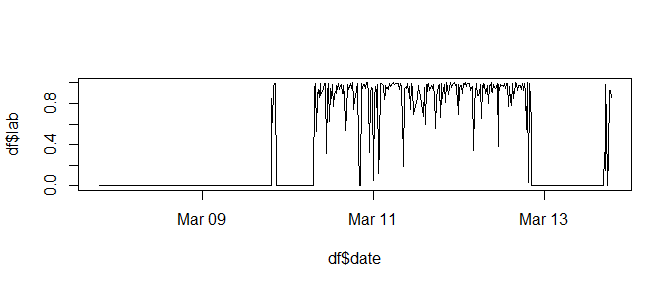Interpretation: The local IAB analysis further corroborates the timing of interactive behaviour observed using the local Prox analysis and the local di statistic. Here we can see that the strongest interactions occur from midday Thursday until Sunday morning. The shape of the local IAB graph is the opposite of that observed with Prox.

### Dynamic Interaction index

Further, the DI statistic provides a spatially and temporally local alternative (di) that can be computed for each pair of simultaneous movement segments. The di index affords the ability to investigate the spatial and temporal dynamics of dynamic interaction behaviour, through plots of di through time, or maps of di. Thus, the local version – di can be said to measure the dynamics of dynamic interaction behaviour. Note, similar to the Cr statistic, DI and di do not consider the distance separating the two individuals, and it is up to the analyst to determine if the necessary conditions exist for interactive behaviour. If option local = TRUE the function returns a dataframe with columns corresponding to the local measures (di, di.theta, and di.d – and time- and/or distance-based weights if set to TRUE). For more detailed information see the documentation, but see also Long and Nelson (2013).Much like with Prox, in order to further examine local level dynamics in the cohesiveness of movement, a time-series plot of di can be used to identify temporal trends in cohesive movement behaviour.

#obtain the local di analysis data-frame
di.df <- DI(deer37, deer38, tc=7.5*60, local=TRUE)
#Examine the temporal dynamics of local di
plot(di.df$date, di.df$di,type="l")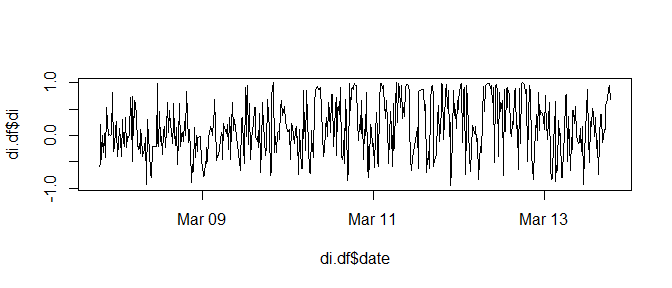Interpretation: Here we see that the time-series plot of di reveals very abrupt fluctuations in di, from low to high values. These fluctuations may suggest little evidence of any periods where sustained cohesive (positive di) or opposing (negative di) movement occurs. In previous analysis, I have found it useful to use a temporal window in order to smooth out the fine-scale fluctuations in di to get a better idea of broader trends. Here I use a 12 hour window in order to re-plot the local level di, and view the dynamic changes in di.

#Smoothed version of local di
di.df$smooth <- 0 #4 fixes/hour x 6 hours on either side of 12 hour centered window w <- 4*6 n <- dim(di.df) #no. of fixes for (i in (w+1):(n-1-w)){ di.temp <- di.df$di[(i-w):(i+w)]
di.df$smooth[i] <- mean(di.temp,na.rm=T) } plot(di.df$date, di.df\$smooth,type="l")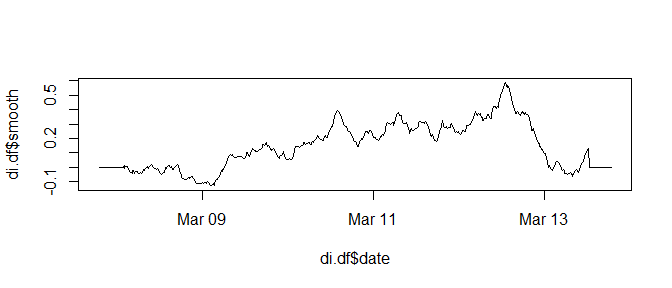From the smoothed time-series plot we can again see a similar pattern as with Prox, whereby cohesive movement behaviour is strongest between mid-day Thursday into early Sunday morning. Within this period there are variations in the cohesive movement behaviour, perhaps related to the diurnal cyclical behaviour associated with deer movements. In situations where periods of cohesive movement are interspersed with random movement, the time-series plot of di (and/or smoothed di) can provide useful insight as to when and/or where this behaviour occurs.

# Summary

In this vignette I have demonstrated how the various methods implemented in the package wildlifeDI can be used to investigate interactive behaviour in wildlife telemetry data. Many of these methods draw on functionality for deriving spatially proximal and temporally simultaneous fixes that are dependent on the critical thresholds $$d_c$$ and $$t_c$$. Thus, care must be taken to ensure the selection of these thresholds as biologically relevant and appropriate with ones dataset (e.g., related to the sampling interval). In this document I have attempted not to argue for or against the use of any of the statistics in different situations. Also, if you are aware of another method for measuring dynamic interaction behaviour feel free to contact me and I will do my best to implement it as I see fit. Finally, thanks for taking the time to utilize these tools and I would appreciate any feedback and/or bugs identified.